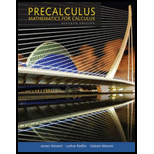Chapter 8.3, Problem 33E### Precalculus: Mathematics for Calcu...

7th Edition
James Stewart + 2 others
ISBN: 9781305071759

#### Solutions

Chapter
Section### Precalculus: Mathematics for Calcu...

7th Edition
James Stewart + 2 others
ISBN: 9781305071759
Textbook Problem

# Polar Form of Complex Numbers Write the complex number in polar form with argument θ between 0 and 2π.33. − 3 − i

To determine

To find: The complex number in polar form for 3i .

Explanation

Given:

The complex number is 3i .

Formula Used:

Formula 1: The formula for the polar form of the complex number is,

z=r(cosθ+isinθ)

Where

•   r is the modulus of z .
• θ is the argument of z .

Formula 2:  The formula for the modulus of z is,

r=a2+b2

Formula 3: The formula for the argument of z is,

θ=tan1(ba)

Calculation:

The complex number is 3i .

Use formula 2 of the modulus of z ,

r=a2+b2

Substitute 3 for a and 1 for b in the above formula,

r=(3)2+(1)2r=3+1r=4r=2

The value of modulus of z is 2

### Still sussing out bartleby?

Check out a sample textbook solution.

See a sample solution

#### The Solution to Your Study Problems

Bartleby provides explanations to thousands of textbook problems written by our experts, many with advanced degrees!

Get Started

## Additional Math Solutions

#### Find more solutions based on key concepts